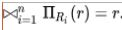Open In App
Related Articles
• Write an Interview Experience
• DBMS Tutorial – Database Management System

# Introduction of 4th and 5th Normal form in DBMS

Prerequisite – Functional Dependency, Database Normalization, Normal Forms

If two or more independent relations are kept in a single relation or we can say multivalue dependency occurs when the presence of one or more rows in a table implies the presence of one or more other rows in that same table. Put another way, two attributes (or columns) in a table are independent of one another, but both depend on a third attribute. A multivalued dependency always requires at least three attributes because it consists of at least two attributes that are dependent on a third.

For a dependency A -> B, if for a single value of A, multiple values of B exist, then the table may have a multi-valued dependency. The table should have at least 3 attributes and B and C should be independent for A ->> B multivalued dependency.

Example:

```Person->-> mobile,
Person ->-> food_likes ```

This is read as “person multi determines mobile” and “person multi determines food_likes.”
Note that a functional dependency is a special case of multivalued dependency. In a functional dependency X -> Y, every x determines exactly one y, never more than one.

## Fourth Normal Form (4NF)

The Fourth Normal Form (4NF) is a level of database normalization where there are no non-trivial multivalued dependencies other than a candidate key. It builds on the first three normal forms (1NF, 2NF, and 3NF) and the Boyce-Codd Normal Form (BCNF). It states that, in addition to a database meeting the requirements of BCNF, it must not contain more than one multivalued dependency.

### Properties

A relation R is in 4NF if and only if the following conditions are satisfied:

```1. It should be in the Boyce-Codd Normal Form (BCNF).
2. The table should not have any Multi-valued Dependency.```

A table with a multivalued dependency violates the normalization standard of the Fourth Normal Form (4NF) because it creates unnecessary redundancies and can contribute to inconsistent data. To bring this up to 4NF, it is necessary to break this information into two tables.

Example: Consider the database table of a class that has two relations R1 contains student ID(SID) and student name (SNAME) and R2 contains course id(CID) and course name (CNAME).

Table R1

Table R2

When their cross-product is done it resulted in multivalued dependencies.

Table R1 X R2

Multivalued dependencies (MVD) are:

` SID->->CID; SID->->CNAME; SNAME->->CNAME`

## Joint Dependency

Join decomposition is a further generalization of Multivalued dependencies. If the join of R1 and R2 over C is equal to relation R then we can say that a join dependency (JD) exists, where R1 and R2 are the decomposition R1(A, B, C) and R2(C, D) of a given relations R (A, B, C, D). Alternatively, R1 and R2 are a lossless decomposition of R. A JD ⋈ {R1, R2, …, Rn} is said to hold over a relation R if R1, R2, ….., Rn is a lossless-join decomposition. The *(A, B, C, D), (C, D) will be a JD of R if the join of joins attribute is equal to the relation R. Here, *(R1, R2, R3) is used to indicate that relation R1, R2, R3 and so on are a JD of R. Let R is a relation schema R1, R2, R3……..Rn be the decomposition of R. r( R ) is said to satisfy join dependency if and only ifJoint Dependency

Example:

Table R1

`Company->->Product `

Table R2

`Agent->->Company `

Table R3

`Agent->->Product `

Table R1⋈R2⋈R3

`Agent->->Product `

## Fifth Normal Form / Projected Normal Form (5NF)

A relation R is in Fifth Normal Form if and only if everyone joins dependency in R is implied by the candidate keys of R. A relation decomposed into two relations must have lossless join Property, which ensures that no spurious or extra tuples are generated when relations are reunited through a natural join.

### Properties

A relation R is in 5NF if and only if it satisfies the following conditions:

```1. R should be already in 4NF.
2. It cannot be further non loss decomposed (join dependency).```

Example – Consider the above schema, with a case as “if a company makes a product and an agent is an agent for that company, then he always sells that product for the company”. Under these circumstances, the ACP table is shown as:

Table ACP

The relation ACP is again decomposed into 3 relations. Now, the natural Join of all three relations will be shown as:

Table R1

Table R2

Table R3

The result of the Natural Join of R1 and R3 over ‘Company’ and then the Natural Join of R13 and R2 over ‘Agent’and ‘Product’ will be Table ACP.

Hence, in this example, all the redundancies are eliminated, and the decomposition of ACP is a lossless join decomposition. Therefore, the relation is in 5NF as it does not violate the property of lossless join